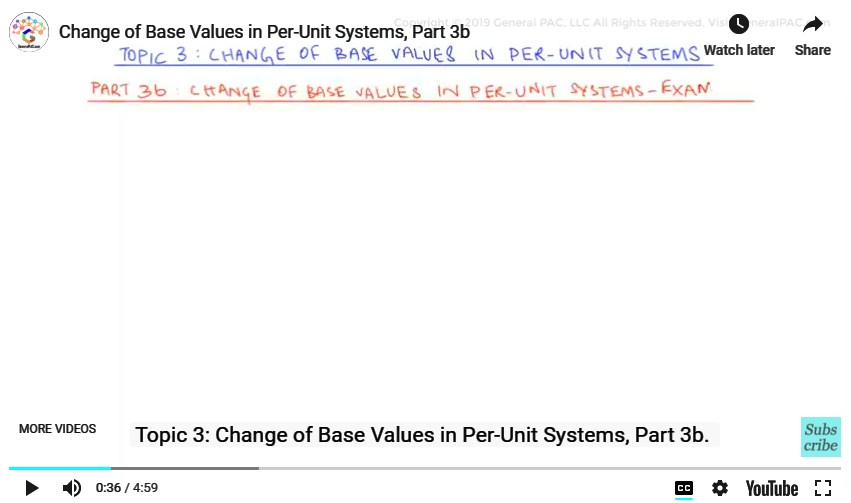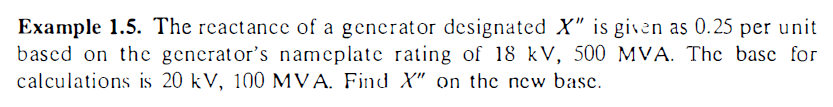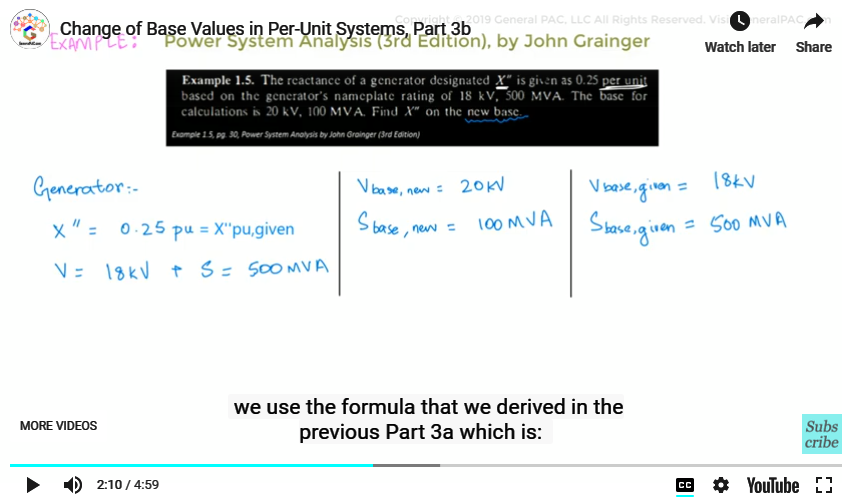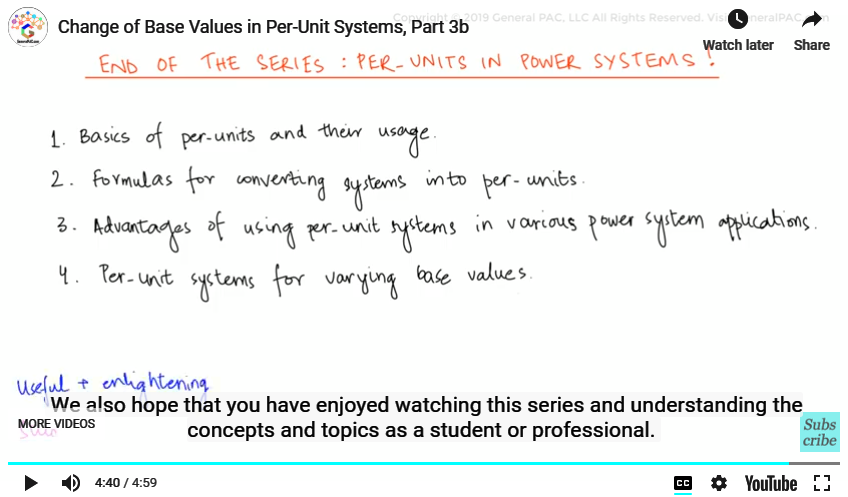This video was brought to you by GeneralPAC.com, making power systems Intuitive, Open and Free for Everyone, Everywhere. Consider subscribing and supporting through patreon.com/GeneralPAC. This is a mechanism for you to support us financially so we can continue making high quality power system video tutorials. Our corporate sponsor for this topic is AllumiaX.com from Seattle, Washington. Contact them for industrial and commercial power system studies.

Change of Base Values in Per-Unit Systems, Part 3b.

In the previous video, Part 3a, we derived a formula that was used in the cases where the base values are changed. In this video Part 3b, we will explain how the formula is employed using a simple example.The example is taken from the book Power System Analysis by John Grainger (3rd Edition).In this example the reactance of the generator is given as:

X'' =0.25 p.u.

And the ratings of the generator are specified as:

V=18 kV

And;

S=500 MVA

The problem asks us to find the reactance of the generator in per-units according to the new base values given. And the new base values are:

Vbase,new = 20 kV

And;

Sbase,new = 100 MVA

Now we can see that the reactance X’’ is specified in per-units in the question. We can comprehend that this value is the given per unit value of the reactance so we can write it as:

X''p.u,given=0.25 p.u.

And we have to calculate the new value for this reactance.

We also know that the rating of the generator given are taken as the base values as we learned in earlier topics of this series. So we can comprehend that the given X’’ value is calculated using the given base values so we can write them as:

Vbase,given = 18 KV

And;

Sbase,given = 500 MVANow for calculating the new value for X’’ in per-units we use the formula we derived in the previous video Part 3a which is:

Zp.u,new = Zp.u,given * (Vbase,given2/ Vbase,new2) * (Sbase,new / Sbase,given)

Or:

X''p.u,new = X''p.u,given * (Vbase,given2/ Vbase,new2) * (Sbase,new / Sbase,given)

Putting the values from the question:

X''p.u,new = 0.25 * ((18 KV)2/ (20 KV)2)) * (1000 MVA / 500 MVA)

Solving the equation:

X''p.u,new = 0.25×0.81×0.2

X''p.u,new = 0.0405 p.u.

So if we compare the new impedance value, we an clearly see that it’s quite a bit smaller than the previous one. We are compareing 0.0405 per unit with our original 0.25 per unit. This makes total sense because previously, the 0.25 per unit impedance was calculated with a considerably larger MVA rating (500 MVA) so when we covered the impedance to a small MVA rating (100 MVA) the effective impedance value decreased considerably.

Here we have the answer to the simple example we solved. This method is employed everywhere where there is a need of calculating new per-unit values if the base values have been changed; whether it is simple power system analysis or a complex one.

This video marks the end of the series of “Per-Unit Systems”. Let’s summarize what we have learned in the 3 topics of this series:

1. Basics of per-unit systems and their usage.
2. Formulas for converting systems into per-units.
3. Advantages of using per-unit systems in various power system applications.
4. Per-unit systems for varying base values.We hope you find this content useful and enlightening. We also hope that you have enjoyed watching this series and understood the concepts of the topics as a student or professional.

For more intuitive video tutorials, please consider subscribing to GeneralPAC.com or becoming a patron on patreon.com/generalpac. GeneralPAC.com. Making Power Systems Intuitive, Open and Free to everyone, everywhere.

Thankyou!

#### Greetings from the GeneralPAC Team!

We make high-quality Power Systems Video Tutorials on complex topics that are free and open to everyone!  Thank you so much for supporting us through Patreon so we can continue doing good and valuable work.

What is Patreon and why do we use it?

Patreon is a fantastic portal that allows our fans and community to make monthly contribution (like Netflix subscription) so we can continue creating high-quality power systems video tutorials. In return, you get access to incredible perks like voting on future topics, getting your questions answered, access to VIP Q/A webinars with the creators of GeneralPAC, and much more! We THANK YOU for supporting us

Why do we need your support?

An incredible amount of time and effort is needed to develop high-quality video tutorials. Each video (Part 1 for example) takes approximately 10 hours to complete which includes learning the concept ourselves, brainstorming creative ways to teach and explain the concepts, writing the script, audio recording, video recording, and editing. It's no wonder why Hundreds-of-Thousands of people have watched, liked, subscribed, and left positive comments on Youtube channel. Your support truly makes all the difference.